MORE IN Finite Element Analysis
MU Mechanical Engineering (Semester 8)
Finite Element Analysis
May 2015
Total marks: --
Total time: --
INSTRUCTIONS
(1) Assume appropriate data and state your reasons
(2) Marks are given to the right of every question
(3) Draw neat diagrams wherever necessary

1 (a) Define a bandwidth of a matrix? What is its significance in FEM? Calculate the band width for the mentioned below F.E. mesh of One Dimensional field problem.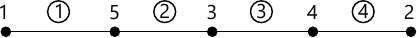10 M
1 (b) Find the weak form and its Quadratic functional for the following given governing Differential Equations: $k \dfrac {d^2u}{dx^2} - \lambda u+2x^2 = 0$ where k, λ are constants, 0
10 M

2 (a) Consider an axial tension problem given in Figure. The bar has a linearly varying cross-sectional area A=(x+1)m2 in the region 0m7 pa. The bar is subjected to a point load P=200 N at x=0.75 m and the bar is constrained at x=0m.
Construct the element stiffness matrix and force matrix. Solve the system of linear equations and find the nodal displacements and elements stresses. Suggest how to improve the finite element model to get more accurate results.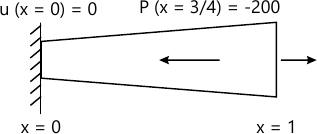10 M
2 (b) Linear Springs are connected to the carts as shown in figure. Imagine that only horizontal displacements are allowed/ Write down the Global Equations and calculate te nodal displacements and reactions at the constraints.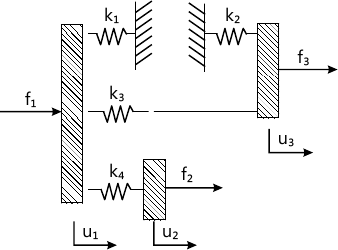10 M

3 (a) Consider a three - noded element in one dimensional Heat Transfer application. The element length is 50cms, with one of the node is located at x=20 cms. The temperatures at the nodes are given by T1=300°C, T2=100°C, T3=60°C. Find the temperature field at x=35 cms
8 M
3 (b) The following governing differential equation represents the flow of a Newtonian viscous fluid on an inclined flat surface. The momentum equations, for a fully developed steady laminar flow along the z coordinate, is given by $-\mu \dfrac {d^2w} {dx^2} = \rho g \cos \beta$ Where w is the z component of the velocity, μ is the viscosity of the fluid, ρ is the density, g is the acceleration due to gravity, and β is the angle between the inclined surface and the vertical. The boundary conditions associated with the problem are that the shear stress is zero at x=0 and the velocity is zero at x=L. $( \dfrac {dw}{dx} \right )\bigg \vert _{x=0}=0; \ w(L)=0$ Formulate the finite element equation (i.e. in terms of the stiffness matrix unknown nodal field vector and load vectors) using Rayleigh Ritz's finite element method approach for a two-noded element of length L with the interpolation functions.
12 M

4 (a) Explain with the help of suitable sketches, about the connectivity conditions pertaining to Primary Variables and Secondary variables at junction modes during assembly.
5 M
4 (b) For the Three Bar plane Truss structure shown in figure. Determine the nodal displacement, stresses in each element and reaction at support by using Multipoint constraint (MPC) method. Take E=207GPa; A=5 cm2, P=103KN (1000 KN); L=100cm.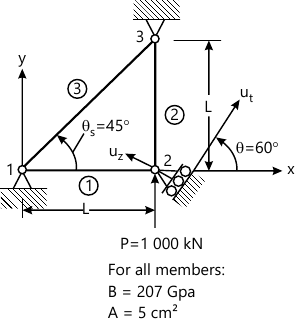15 M

5 (a) (i) Explain Constant strain triangle (CST).
2 M
5 (a) (ii) Derive 2-D quadratic interpolation shape functions for a triangular element in terms of Area Coordinates.
8 M
5 (b) For the iso-parametric quadrilateral elements shown in Figure. Determine (a) Cartesian coordinates of the point P which has local coordinates (ξ=0.57735 and η=0.57735)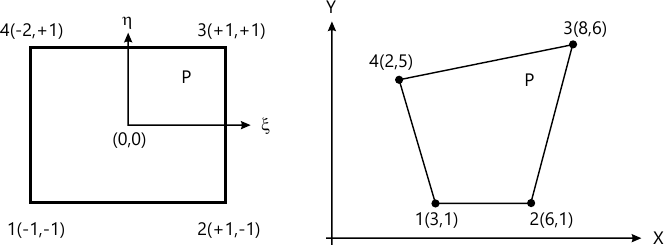10 M

6 (a) Discuss briefly higher order and iso-parametric elements with suitable sketches.
5 M
6 (b) Find the natural frequencies of longitudinal vibrations of the constrained stepped shaft of areas A and 2A and of equal lengts (L), as shown below. Compare the results obtained using lumped mass matrix approach.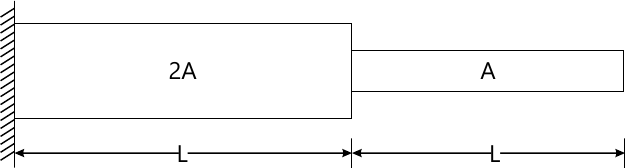15 M

7 (a) Evaluate the following intergral using Gausian Legendre Quadrature method for the integral and compare with exact result. $I=\int^3_0 \int^2_1 \ x \ y (1+x+y)dx \ dy$
 7 ξi Wi 1 0.0 2.0 2 ±0.5774 1.0 3 0.0 ±0.7746 0.8889 0.556
10 M
7 (b) Solve the following differential equation by using any of the Two Method i) Galerian ii) Least Squares iii) Sub Domain iv) Collocation. \[ - \dfrac {d^2u}{dx^2} + 2 \ x^2 = 0, 0 Compare your answer with exact at minimum three points within the domain.
10 M

More question papers from Finite Element Analysis